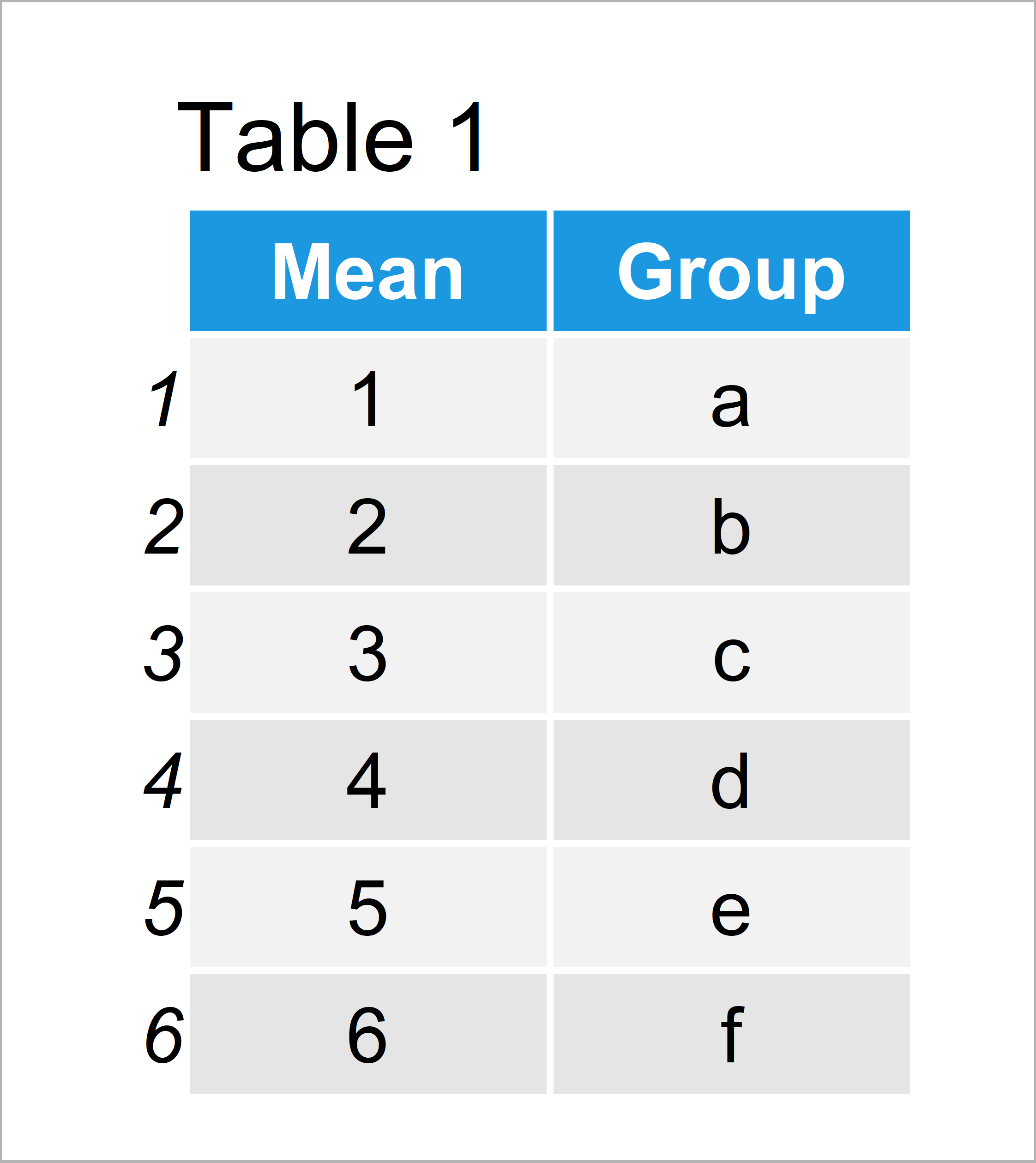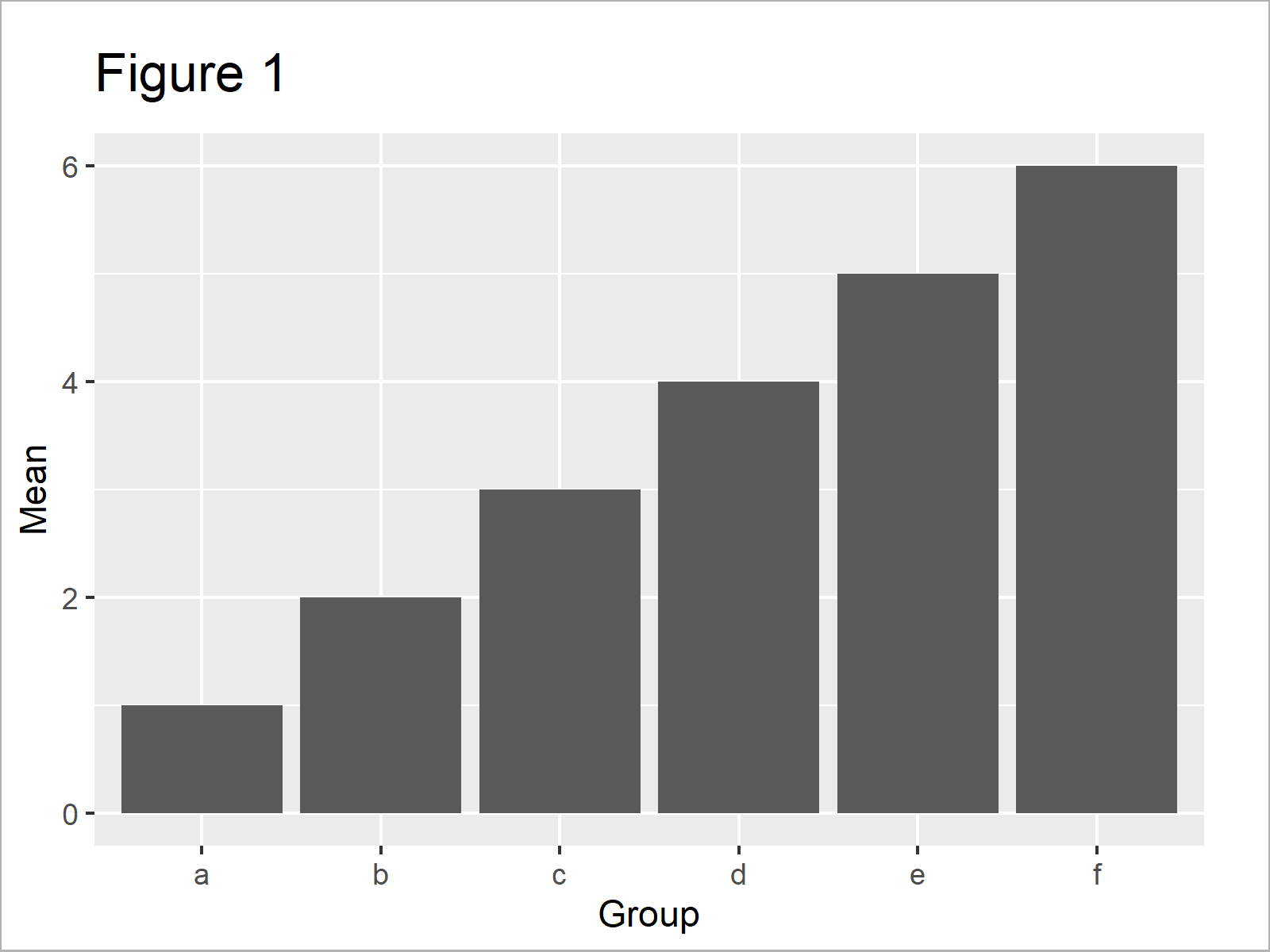# R ggplot2 Error: Don’t know how to automatically pick scale for object type

This article illustrates how to deal with the ggplot2 error “Don’t know how to automatically pick scale for object type” in R.

The tutorial contains this information:

Let’s dig in!

## Example Data & Add-On Packages

Consider the following example data.

```data <- data.frame(Mean = 1:6,      # Create example data
Group = letters[1:6])
data                                # Print example data```Table 1 reveals the structure of our example data: It contains six rows and the two columns “Mean” and “Group”.

In order to plot our data using the ggplot2 package, we also need to install and load ggplot2:

```install.packages("ggplot2")         # Install ggplot2 package

## Example 1: Reproduce the ggplot2 Error – automatically pick scale for object of type standardGeneric

In this example, I’ll illustrate how to replicate the ggplot2 error “Don’t know how to automatically pick scale for object type” in R.

Let’s assume that we want to draw our data in a ggplot2 barplot. Then, we might try to use the following R code:

```ggplot(data, aes(Group, mean)) +    # ggplot function leads to error
geom_bar(stat = "identity")
# Don't know how to automatically pick scale for object of type standardGeneric. Defaulting to continuous.
# Error: Aesthetics must be valid data columns. Problematic aesthetic(s): y = mean.
# Did you mistype the name of a data column or forget to add after_stat()?```

Unfortunately, the R programming language returns the ggplot2 error “Don’t know how to automatically pick scale for object type”.

The reason for this is that we have specified the y variable to be equal to “mean” instead of “Mean” (note the lower/upper case of the “M”). For that reason, R assumes that we want to use the mean function instead of our Mean variable – which is not possible.

So how can we solve this problem? That’s what I’ll explain next.

## Example 2: Fix the ggplot2 Error – automatically pick scale for object of type standardGeneric

This example shows how to avoid the ggplot2 error message “Don’t know how to automatically pick scale for object type”.

For this, we simply need to specify the variable name of our data properly (i.e. upper case “Mean):

```ggplot(data, aes(Group, Mean)) +    # ggplot function draws plot properly
geom_bar(stat = "identity")```The output of the previous R syntax is shown in Figure 1: A ggplot2 barchart. The error message does not appear anymore.

## Video & Further Resources

Would you like to learn more about the ggplot2 package and its variety of functions, then please check out the video below, where you get a detailed introduction to the package and data visualization in R.

Please accept YouTube cookies to play this video. By accepting you will be accessing content from YouTube, a service provided by an external third party.If you accept this notice, your choice will be saved and the page will refresh.

Also, you may read the other RStudio articles on this website:

In this article, I have explained how to handle the ggplot2 error message “Don’t know how to automatically pick scale for object type” in R.

Note that the ggplot2 package sometimes returns a very similar error message when other functions are set as input within the ggplot or aes function.

For instance, the following error message appears when we specify the sample function as input: “Don’t know how to automatically pick scale for object of type function. Defaulting to continuous.”.

However, the explanations of this tutorial can also be used to fix this error message in R.

In case you have further questions, let me know in the comments.

Subscribe to the Statistics Globe Newsletter